Reference hyper-netted chain

The reference hyper-netted chain (RHNC) is sometimes referred to as the modified-HNC (MHNC) (Ref. 1) Rosenfeld and Ashcroft (1979) (Ref. 1) proposed the `anzatz of universality':

"...the bridge functions.. ..constitute the same family of curves, irrespective of the assumed pair potential"

The basis of the method is to solve the modified HNC equation (with inclusion of the one-parameter bridge functions appropriate to hard spheres), and determine the only free parameter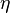$\eta$ (related to the hard-sphere diameter) by requiring thermodynamic consistency. Fred Lado (Ref. 2) and Rosenfeld and Ashcroft (1979) (Ref. 3) noticed that the Ornstein-Zernike relation can always be written in the form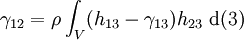$\gamma_{12} = \rho \int_V (h_{13} - \gamma_{13}) h_{23} ~{\rm d}(3)$

In view of this a hybrid solution between the hyper-netted chain approximation and the Malijevsky-Labik formula for hard spheres (Ref. 4 and 5) was proposed. The philosophy of this method is that the bridge functional of the liquid is fairly similar to that of the hard sphere fluid. (See also Ref.s 6 and 7) The RHNC closure is given by (Eq. 17 Ref. 7)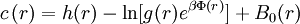$c\left(r\right) = h(r) - \ln [g(r) e^{\beta \Phi(r)}] + B_0(r)$

along with the constraint (Eq. 18 Ref. 7)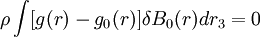$\rho \int [g(r) - g_0(r)] \delta B_0(r) dr_3 = 0$

where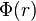$\Phi(r)$ is the intermolecular pair potential. Incorporating a reference potential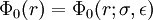$\Phi_0(r)= \Phi_0(r;\sigma,\epsilon)$ this equation becomes (Eqs. 19a and 19b in Ref. 7)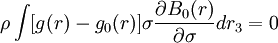$\rho \int [g(r) - g_0(r)] \sigma \frac{\partial B_0(r)}{\partial \sigma}dr_3 = 0$

and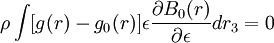$\rho \int [g(r) - g_0(r)] \epsilon \frac{\partial B_0(r)}{\partial \epsilon} dr_3 = 0$

These are the conditions that will determine the optimum values of$\sigma$ and$\epsilon$ that minimise the free energy. The RHNC satisfies the 'Hiroike' termodynamic relation test Ref. 7 and 9, i.e.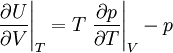$\left.\frac{\partial U}{\partial V}\right\vert_{T} = T \left.\frac{\partial p}{\partial T}\right\vert_{V} -p$

For highly asymmetric mixtures see Ref. 9.